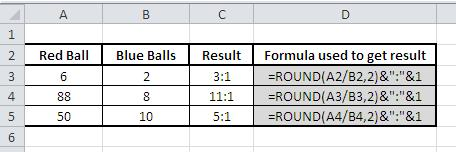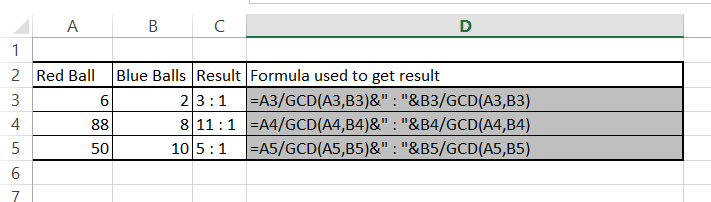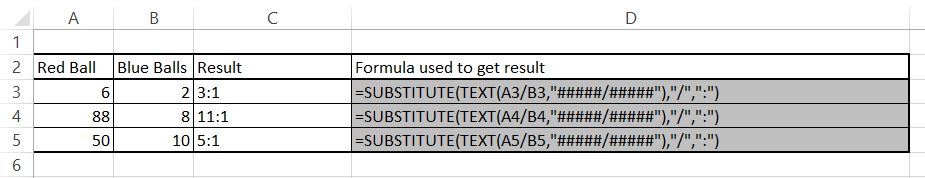#### How to calculate ratio?

In mathematics, a ratio is a comparison of two numbers. It is expressed as a colon (:) between the two numbers. For example, if there are 2 red balls and 3 blue balls on a table, the ratio of red balls to blue balls is 2:3.

## How to calculate ratio in Excel

There are three ways to calculate ratio in Excel:

Method Formula Example
ROUND function =ROUND(A3/B3, 2) =ROUND(2/3, 2) = 0.67
GCD function =A3/GCD(A3, B3) & ” : ” & B3/GCD(A3, B3) =2/GCD(2, 3) & ” : ” & 3/GCD(2, 3) = 2/1 : 3/1
Text function method =SUBSTITUTE(TEXT(A3/B3, “#####/#####”), “/”, “:”) =SUBSTITUTE(TEXT(2/3, “#####/#####”), “/”, “:”) = “2:3”

## Using the ROUND functionThe ROUND function in Excel is used to trim down (reduce) a given value to a specific number of decimal places.

= ROUND (Number, Num_digits )

The ROUND function will round up or down depending on whether the last digit is greater than or less than 5.

## Using the GCD function

There is also a method using the GCD function. GCD stands for Greatest Common Divisor.

Finding the Greatest Common Divisor is the basis of the formula. To calculate the ratio, you just need to divide two numbers by their GCDs.

The formula I used in the example is:

=A3/GCD(A3,B3)&” : “&B3/GCD(A3,B3)Calculating the ratio using the GCD function is the most convenient method.

## Using text functions

There is also a way to calculate ratio in Excel using text functions. However, this method is not as commonly used as the other two methods.